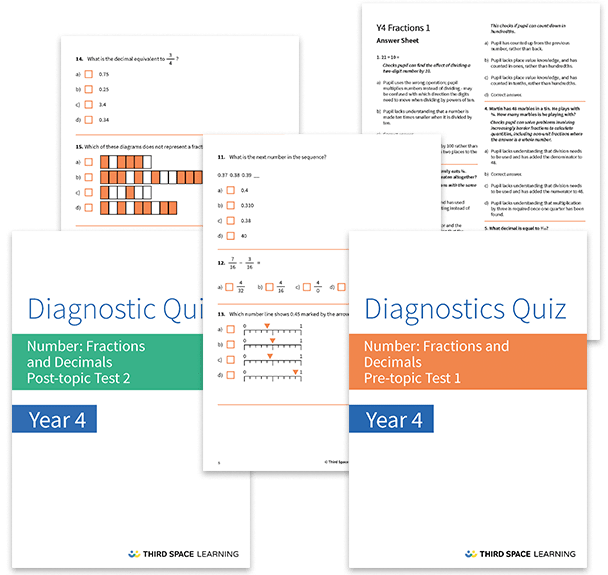# Fractions and Decimals Diagnostic Assessments Year 4

This pack of resources contains two diagnostic assessments for fractions and decimals, one pre and one post topic assessment. Each test contains 24 multiple choice questions with detailed answers that not only indicate the correct answer but also explain the distractor answers. You can use these tests to help you diagnose specific misconceptions and learning gaps before starting a block of learning and after the block has been completed.

These tests cover:

• Dividing by 10
• Adding and subtracting fractions with the same denominator (including beyond one whole)
• Counting in fractions including hundredths
• Finding fractions of amounts
• Identifying decimal equivalents of tenths, hundredths and ¼, ½, ¾
• Identifying equivalent fractions
• Identifying the value of digits as ones, tenths or hundredths
• Comparing numbers with two decimal places
• Rounding decimals to the nearest whole number
• Adding decimals in the form of money

Year 4

Fractions, Decimals